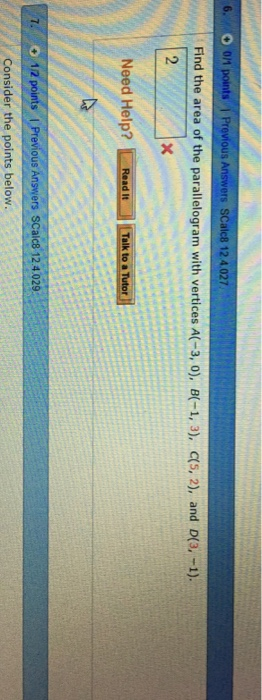# Find the area of the parallelogram with vertices a(−3. 0). b(−1. 3). c(5. 2). and d(3. −1).Find the area of the parallelogram with vertices A(-3, 0), B(-1, 3), c(5, 2), and D(3, -1). 2 Consider the points below.

The area of the parallelogram with the vertices A:(-3,0),
B;(-1,3),C:(5,2),D:(3,-1) is ||DC|x|AD||. Now DC = (3,-1)-(5,2) =
(-2,-3) and AD = (3,-1)-(-3,0) = (6,-1). Therefore, DC x AD = | i
j k |

|-2 -3 0 |

| 6 -1 0 |
= i( -3*0-0*-1)-j(-2*0-0*6)+k(-2*-1-6*-3) = k(2+18) = 0i +0
j+20k.
Hence the area of the parallelogram ABCD is |0i +0 j+20k
| = √(02 +02 +202) = √400 = 20.